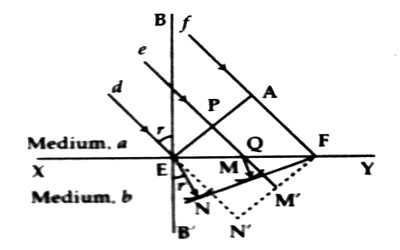# Laws of Refraction in the light of Huygens’s Principle

Laws of Refraction in the light of Huygens’s Principle

According to Huygens’s principle, any point in the wavefront can be considered as the source of spherical waves. We can state the Huygens’s principle in the following way.

Statement: Each point on any wavefront is considered as the source of oscillation or disturbance. The waves generated from those secondary sources progress forward with the same velocity of the principal wave. It a surface is drawn by the tangents of those secondary waves at any time, then that surface will represent the new position of the wavefront at that time.

Huygens’s Principle provides a convenient way to visualize refraction. If points on the wavefront at the boundary of a different medium serve as sources for the propagating light, one can see why the direction of the light propagation changes.

Let ‘a’ and ‘b’ be two transparent isotropic media. Let XY be the surface of separation between the two media. The velocities of light in the medium ‘a’ is va and that in the medium ‘b’ is vb. Here va > vb.Fig: Refraction in the light

Suppose d, e, f are three parallel rays. These rays are incident obliquely on the XY plane [Figure]. APE is the wavefront of the rays. Let the wavefront EPA first touch the point E of the surface of separation. According to the Huygens’s principle, the particle at the point E being disturbed produces a secondary wave and spread with velocities va and vb, in the media ‘a’ and  ‘b’ respectively. Now, if ‘t’ be the time taken to reach the disturbance from point A to point F, then FA = vat. At that time the light wave from the point E in medium ‘b’ will travel the distance EN. So, EN = vbt. With A as a center and EN = vat as radius, let an arc be drawn and if a tangent FN and it is drawn, then FMN will indicate the wavefront of the refracted ray.

Proof of the laws of refraction:

Let a normal BFBˊ be drawn on XY through the point E.

Now, ∠dEB + ∠BEA = ∠BEA + ∠AEF = 1 right angle

then, ∠dEB = ∠AEF = angle of incidence, ∠i.

Again, ∠BˊEN + ∠NEF = ∠NEF + ∠EFN = 1 right angle

Then, ∠BˊEN = ∠EFN = angle of refraction, ∠r

So, (sin i / sin r) = [(sin ∠dEB) / (sin ∠BˊEN)] = (Sin ∠AEF) / (Sin ∠EFN)

= (AF/EF) / (EN/EF) = AF/EN = vat / vbt = va/vb = a constant = aµb

aµb is the refractive index of the medium ‘b’ with respect to the medium ‘a’.

So, Snell’s law or second law of refraction is proved by it.

Again, the incident ray dE, the refracted ray EN and the normal BEB́ drawn at the point of incidence are on the same plane of the paper. The first law of refraction is proved by it. So, the two laws of refraction are proved on the basis of the wave theory of light.